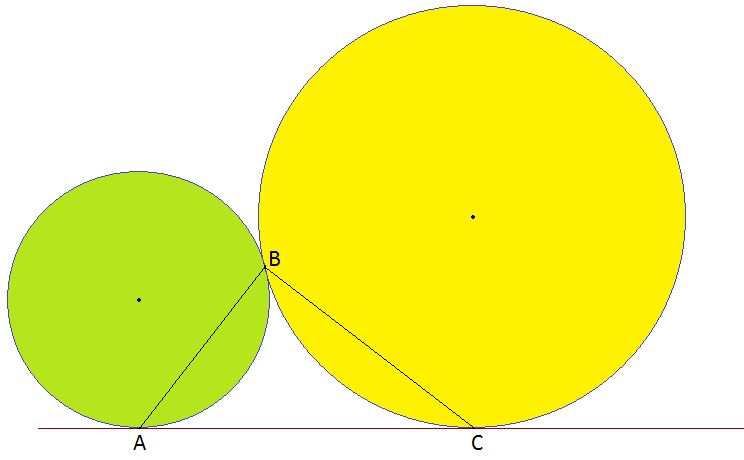Two Circles and a Triangle

Geometry Level 2In the figure $AC$ is a common tangent of two touching circles. If $B$ is the touching point of both circles then which is of the following is true about $\angle ABC$?

×